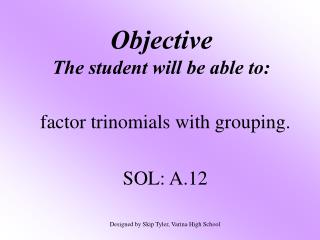# Objective The student will be able to: - PowerPoint PPT PresentationDownload PresentationObjective The student will be able to:

Objective The student will be able to:
Download Presentation## Objective The student will be able to:

- - - - - - - - - - - - - - - - - - - - - - - - - - - E N D - - - - - - - - - - - - - - - - - - - - - - - - - - -
##### Presentation Transcript

1. ObjectiveThe student will be able to: factor trinomials with grouping. SOL: A.12 Designed by Skip Tyler, Varina High School

2. Factoring ChartThis chart will help you to determine which method of factoring to use.TypeNumber of Terms 1. GCF 2 or more 2. Diff. Of Squares 2 3. Trinomials 3

3. Review: (y + 2)(y + 4) y2 First terms: Outer terms: Inner terms: Last terms: Combine like terms. y2 + 6y + 8 +4y +2y y2 +2y +8 +4y +8 In this lesson, we will begin with y2 + 6y + 8 as our problem and finish with (y + 2)(y + 4) as our answer.

4. Here we go! 1) Factor y2 + 6y + 8Use your factoring chart. Nope! Do we have a GCF? Is it a Diff. of Squares problem? Now we will learn Trinomials! You will set up a table with the following information. No way! 3 terms! Product of the first and last coefficients Middlecoefficient The goal is to find two factors in the first column that add up to the middle term in the second column. We’ll work it out in the next few slides.

5. M A Product of the first and last coefficients Middlecoefficient 1) Factor y2+6y + 8Create your MAMA table. Multiply Add+8 +6 Here’s your task… What numbers multiply to +8 and add to +6? If you cannot figure it out right away, write the combinations.

6. 1) Factor y2 + 6y + 8Place the factors in the table. Multiply Add+8 +6 +9, NO -9, NO +6, YES!! -6, NO Which has a sum of +6? +1, +8 -1, -8 +2, +4 -2, -4 We are going to use these numbers in the next step!

7. Multiply Add+8 +6 1) Factor y2 + 6y + 8 +6, YES!! Hang with me now! Replace the middle number of the trinomial with our working numbers from the MAMA table y2+ 6y + 8 y2+ 2y + 4y + 8 Now, group the first two terms and the last two terms. +2, +4

8. We have two groups!(y2+ 2y)(+4y + 8) If things are done right, the parentheses should be the same. Factor out the GCF’s. Write them in their own group. Almost done! Find the GCF of each group and factor it out. y(y + 2) +4(y + 2) (y + 4)(y + 2) Tadaaa! There’s your answer…(y + 4)(y + 2)You can check it by multiplying. Piece of cake, huh?There is a shortcut for some problems too! (I’m not showing you that yet…)

9. M A Product of the first and last coefficients Middlecoefficient 2) Factor x2 – 2x – 63Create your MAMA table. Multiply Add-63 -2 -62 62 -18 18 -2 2 Signs need to be different since number is negative. -63, 1 -1, 63 -21, 3 -3, 21 -9, 7 -7, 9

10. Replace the middle term with our working numbers.x2 – 2x – 63 x2– 9x + 7x – 63 Group the terms. (x2 – 9x) (+ 7x – 63) Factor out the GCF x(x – 9) +7(x – 9) The parentheses are the same! Weeedoggie! (x + 7)(x – 9)

11. Here are some hints to help you choose your factors in the MAMA table. 1) When the last term is positive, the factors will have the same sign as the middle term. 2) When the last term is negative, the factors will have different signs.

12. M A Product of the first and last coefficients Middlecoefficient 2) Factor 5x2 - 17x + 14Create your MAMA table. Multiply Add+70 -17 -71 -37 -17 -1, -70 -2, -35 -7, -10 Signs need to be the same as the middle sign since the product is positive. Replace the middle term. 5x2– 7x – 10x + 14 Group the terms.

13. (5x2– 7x) (– 10x + 14) Factor out the GCF x(5x – 7) -2(5x – 7) The parentheses are the same! Weeedoggie! (x – 2)(5x – 7) Hopefully, these will continue to get easier the more you do them.

14. Factor x2 + 3x + 2 • (x + 2)(x + 1) • (x – 2)(x + 1) • (x + 2)(x – 1) • (x – 2)(x – 1)

15. Factor 2x2 + 9x + 10 • (2x + 10)(x + 1) • (2x + 5)(x + 2) • (2x + 2)(x + 5) • (2x + 1)(x + 10)

16. Factor 6y2 – 13y – 5 • (6y2 – 15y)(+2y – 5) • (2y – 1)(3y – 5) • (2y + 1)(3y – 5) • (2y – 5)(3y + 1)

17. 2) Factor 2x2 - 14x + 12 Multiply Add+6 -7 Signs need to be the same as the middle sign since the product is positive. Find the GCF! 2(x2 – 7x + 6) Now do the MAMA table! -1, -6 -2, -3 -7 -5 Replace the middle term. 2[x2– x – 6x + 6] Group the terms.

18. 2[(x2– x)(– 6x + 6)] Factor out the GCF 2[x(x – 1) -6(x – 1)] The parentheses are the same! Weeedoggie! 2(x – 6)(x – 1) Don’t forget to follow your factoring chart when doing these problems. Always look for a GCF first!!